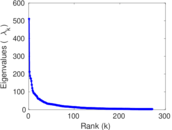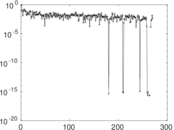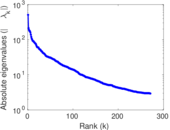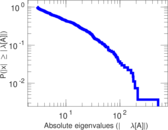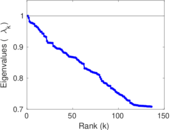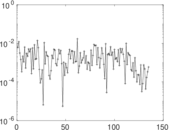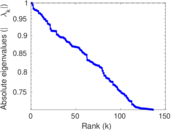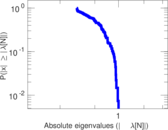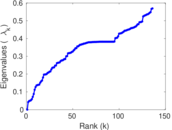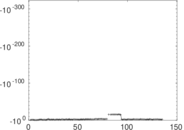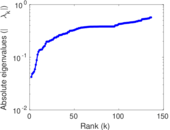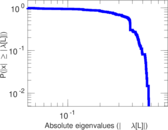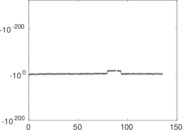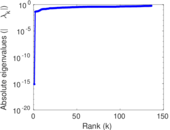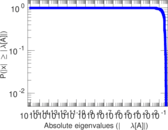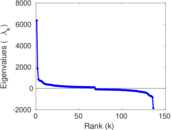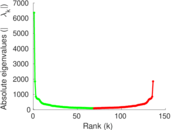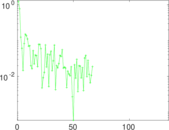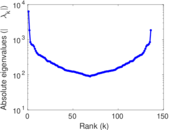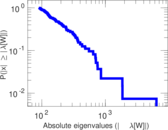# Wikipedia edits (jbo)

This is the bipartite edit network of the Lojban Wikipedia. It contains users and pages from the Lojban Wikipedia, connected by edit events. Each edge represents an edit. The dataset includes the timestamp of each edit.

 Code `jbo` Internal name `edit-jbowiki` Name Wikipedia edits (jbo) Data source http://dumps.wikimedia.org/ AvailabilityDataset is available for download Consistency checkDataset passed all tests Category Authorship network Dataset timestamp 2017-10-20 Node meaning User, article Edge meaning Edit Network formatBipartite, undirected Edge typeUnweighted, multiple edges Temporal dataEdges are annotated with timestamps

## Statistics

 Size n = 6,365 Left size n1 = 908 Right size n2 = 5,457 Volume m = 100,039 Unique edge count m̿ = 44,485 Wedge count s = 15,562,394 Claw count z = 4,897,746,065 Cross count x = 1,376,477,438,949 Square count q = 77,287,790 4-Tour count T4 = 680,663,374 Maximum degree dmax = 7,477 Maximum left degree d1max = 7,477 Maximum right degree d2max = 325 Average degree d = 31.434 1 Average left degree d1 = 110.175 Average right degree d2 = 18.332 2 Fill p = 0.008 977 88 Average edge multiplicity m̃ = 2.248 83 Size of LCC N = 5,678 Diameter δ = 13 50-Percentile effective diameter δ0.5 = 3.350 18 90-Percentile effective diameter δ0.9 = 4.794 51 Median distance δM = 4 Mean distance δm = 3.731 01 Gini coefficient G = 0.839 313 Balanced inequality ratio P = 0.167 870 Left balanced inequality ratio P1 = 0.078 279 5 Right balanced inequality ratio P2 = 0.195 744 Relative edge distribution entropy Her = 0.789 222 Power law exponent γ = 1.866 34 Tail power law exponent γt = 1.611 00 Tail power law exponent with p γ3 = 1.611 00 p-value p = 0.000 00 Left tail power law exponent with p γ3,1 = 1.581 00 Left p-value p1 = 0.000 00 Right tail power law exponent with p γ3,2 = 6.001 00 Right p-value p2 = 0.072 000 0 Degree assortativity ρ = −0.051 786 4 Degree assortativity p-value pρ = 8.326 36 × 10−28 Spectral norm α = 510.363 Algebraic connectivity a = 0.042 258 8 Spectral separation |λ1[A] / λ2[A]| = 2.394 81 Controllability C = 4,574 Relative controllability Cr = 0.725 571

## Plots

### Fruchterman–Reingold graph drawing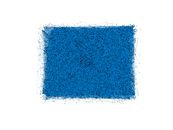### Degree distribution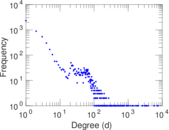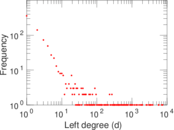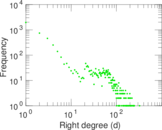### Cumulative degree distribution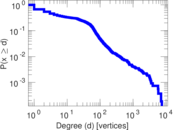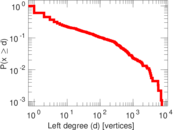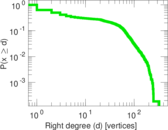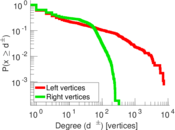### Lorenz curve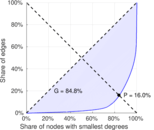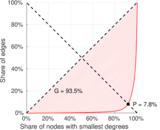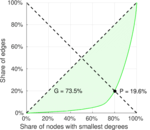### Spectral distribution of the adjacency matrix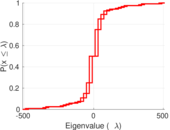### Spectral distribution of the normalized adjacency matrix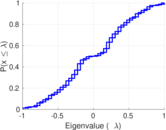### Spectral distribution of the Laplacian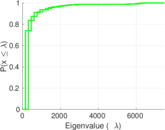### Spectral graph drawing based on the adjacency matrix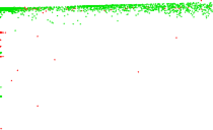### Spectral graph drawing based on the Laplacian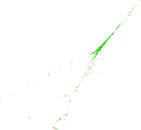### Spectral graph drawing based on the normalized adjacency matrix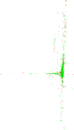### Degree assortativity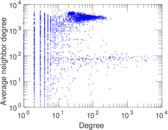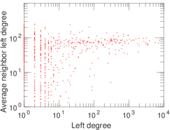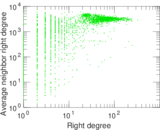### Zipf plot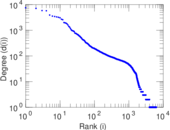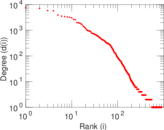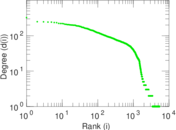### Hop distribution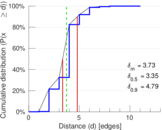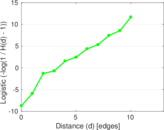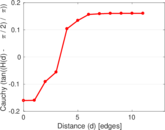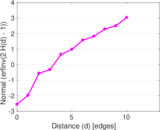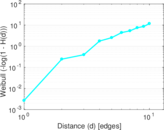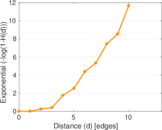### Double Laplacian graph drawing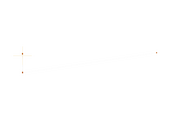### Delaunay graph drawing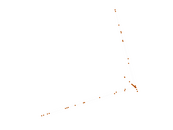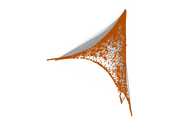### Edge weight/multiplicity distribution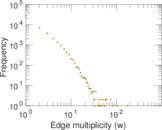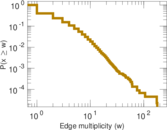### Temporal distribution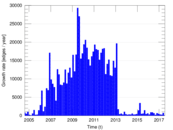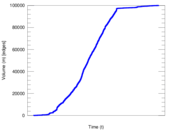### Temporal hop distribution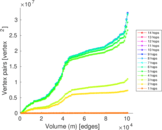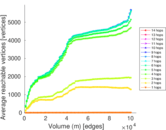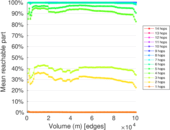### Diameter/density evolution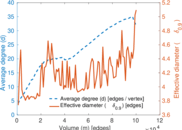### Matrix decompositions plots